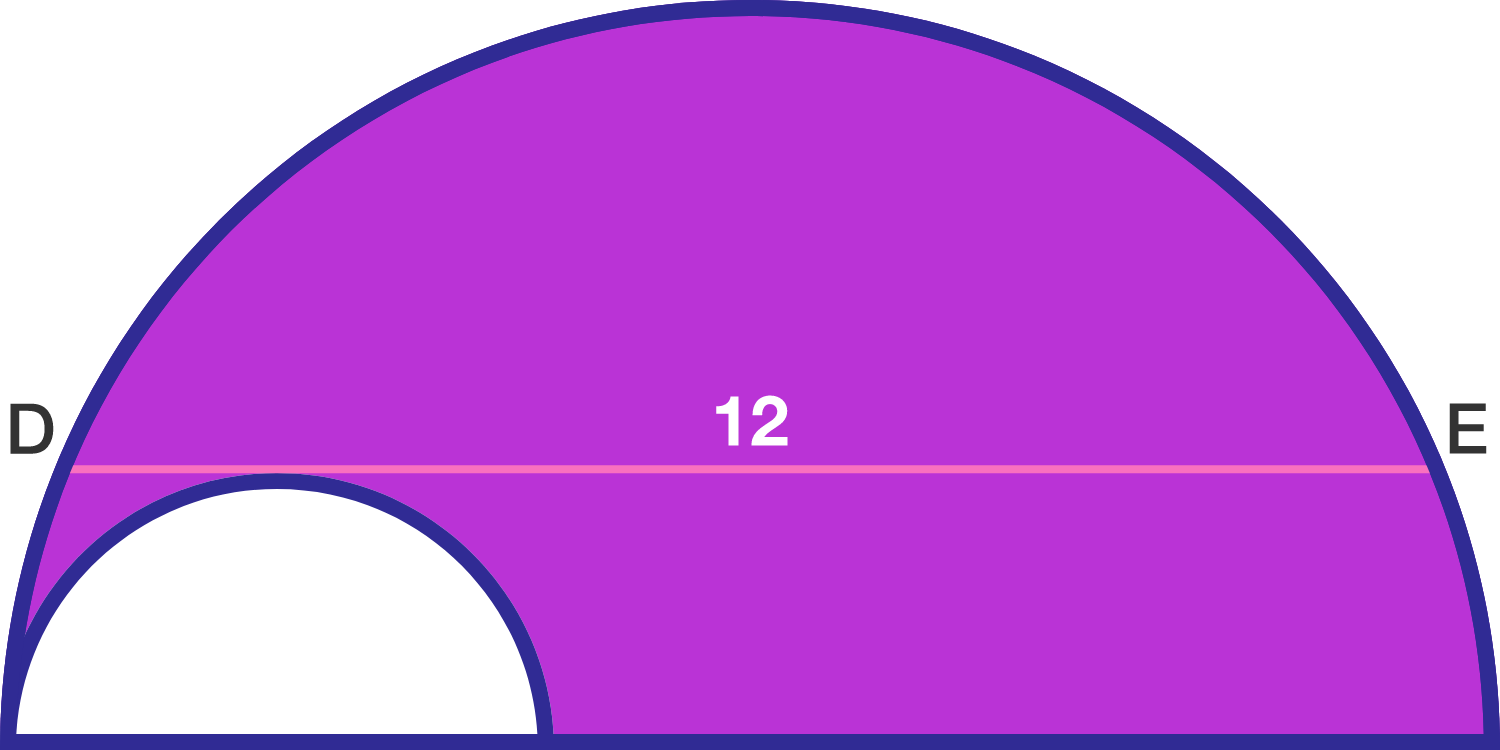Geometry Level 2The figure shows two semicircles whose diameters lie on a common line. The chord $DE$ of the larger circle is parallel to the diameter, tangent to the smaller circle, and has length $12$.

If the purple area is $A \pi$, what is $A$?

×